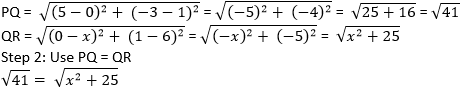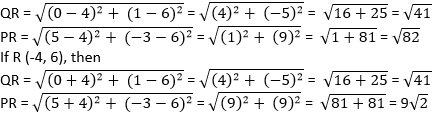Newbie

# If Q (0, 1) is equidistant from P (5, – 3) and R (x, 6), find the values of x. Also find the distance QR and PR. Q.9

• 0

What is the best solution of coordinate geometry of class 10 and how i solve  exercise 7.1 question  .Sir please help me to solve this question easily . If Q (0, 1) is equidistant from P (5, – 3) and R (x, 6), find the values of x. Also find the distance QR and PR.

Share

1. Given: Q (0, 1) is equidistant from P (5, – 3) and R (x, 6), which means PQ = QR

Step 1: Find the distance between PQ and QR using distance formula,Squaring both the sides, to omit square root

41 = x+ 25

x= 16

x = ± 4

x = 4 or x = -4

Coordinates of Point R will be R (4, 6) or R (-4, 6),

If R (4, 6), then QR• 0